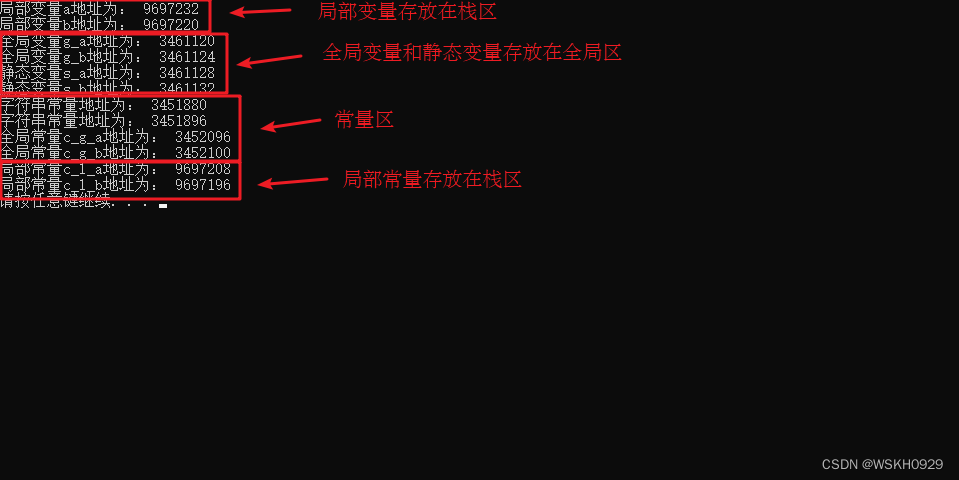## 【C++】从0到1入门C++编程学习笔记 - 核心编程篇：内存分区模型

news/2023/6/6 23:20:01

### 文章目录

• 一、程序运行前
• 二、程序运行后
• 三、new 操作符

C++程序在执行时，将内存大方向划分为4个区域

• 代码区：存放函数体的二进制代码，由操作系统进行管理的
• 全局区：存放全局变量和静态变量以及常量
• 栈区：由编译器自动分配释放, 存放函数的参数值,局部变量等
• 堆区：由程序员分配和释放,若程序员不释放,程序结束时由操作系统回收

# 一、程序运行前

​ 存放 CPU 执行的机器指令

​ 代码区是共享的，共享的目的是对于频繁被执行的程序，只需要在内存中有一份代码即可

​ 代码区是只读的，使其只读的原因是防止程序意外地修改了它的指令

​ 全局变量和静态变量存放在此.

​ 全局区还包含了常量区, 字符串常量和其他常量也存放在此

``````//全局变量
int g_a = 10;
int g_b = 10;//全局常量
const int c_g_a = 10;
const int c_g_b = 10;int main() {//局部变量int a = 10;int b = 10;//打印地址cout << "局部变量a地址为： " << (int)&a << endl;cout << "局部变量b地址为： " << (int)&b << endl;cout << "全局变量g_a地址为： " <<  (int)&g_a << endl;cout << "全局变量g_b地址为： " <<  (int)&g_b << endl;//静态变量static int s_a = 10;static int s_b = 10;cout << "静态变量s_a地址为： " << (int)&s_a << endl;cout << "静态变量s_b地址为： " << (int)&s_b << endl;cout << "字符串常量地址为： " << (int)&"hello world" << endl;cout << "字符串常量地址为： " << (int)&"hello world1" << endl;cout << "全局常量c_g_a地址为： " << (int)&c_g_a << endl;cout << "全局常量c_g_b地址为： " << (int)&c_g_b << endl;const int c_l_a = 10;const int c_l_b = 10;cout << "局部常量c_l_a地址为： " << (int)&c_l_a << endl;cout << "局部常量c_l_b地址为： " << (int)&c_l_b << endl;system("pause");return 0;
}
``````• C++中在程序运行前分为全局区和代码区
• 代码区特点是共享和只读
• 全局区中存放全局变量、静态变量、常量
• 常量区中存放 const修饰的全局常量 和 字符串常量

# 二、程序运行后

​ 由编译器自动分配释放, 存放函数的参数值,局部变量等

​ 注意事项：不要返回局部变量的地址，栈区开辟的数据由编译器自动释放

``````int * func()
{int a = 10;return &a;
}int main() {int *p = func();cout << *p << endl;cout << *p << endl;system("pause");return 0;
}
``````

​ 由程序员分配释放,若程序员不释放,程序结束时由操作系统回收

​ 在C++中主要利用new在堆区开辟内存

``````int* func()
{int* a = new int(10);return a;
}int main() {int *p = func();cout << *p << endl;cout << *p << endl;system("pause");return 0;
}
``````

• 堆区数据由程序员管理开辟和释放

• 堆区数据利用new关键字进行开辟内存

# 三、new 操作符

​ C++中利用new操作符在堆区开辟数据

​ 堆区开辟的数据，由程序员手动开辟，手动释放，释放利用操作符 delete

​ 语法：` new 数据类型`

​ 利用new创建的数据，会返回该数据对应的类型的指针

``````int* func()
{int* a = new int(10);return a;
}int main() {int *p = func();cout << *p << endl;cout << *p << endl;//利用delete释放堆区数据delete p;//cout << *p << endl; //报错，释放的空间不可访问system("pause");return 0;
}
``````

``````//堆区开辟数组
int main() {int* arr = new int;for (int i = 0; i < 10; i++){arr[i] = i + 100;}for (int i = 0; i < 10; i++){cout << arr[i] << endl;}//释放数组 delete 后加 []delete[] arr;system("pause");return 0;
}``````

### （三）CDA 数据分析师Level1考试新版大纲解析（自己整理）PART 3 数据库应用

PART 3 数据库应用 &#xff08;占比 17%&#xff09; 总体要求 理解数据库的基本概念、理解 DDL 及 DML 语言、能够根据业务需求及数据特征使用查询语言从数据库中获取准确、完整的数据信息、能够应用数据库函数进行数据处理及计算 1、数据库相关概念&#xff08;占比 1%&…

### ASKING FOR AND GIVING OPINIONS (from www.globalenglish.com)

Asking for Opinions We use these expressions when we want to ask what another person thinks about something. All these questions are followed by a noun or a noun phrase. How do you feel about the new television show?What do you think of my new computer?

### Some Tips of Gift Giving

Some Tips of Gift Giving Some Tips of Gift GivingGifts are often given to express gratitude, friendship or hospitality. It’s a common courtesy observed in many cultures. Whenever someone receives a present, treat or invitation from a friend, they will try